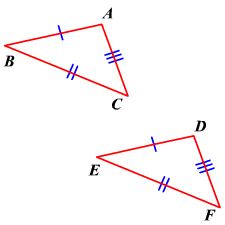# SSS (Side-Side-Side) Postulate

If the three sides of a triangle are congruent to the three sides of another triangle, then the two triangles are congruent.In the figure, $\stackrel{¯}{AB}\cong \stackrel{¯}{DE},\text{\hspace{0.17em}}\text{\hspace{0.17em}}\stackrel{¯}{BC}\cong \stackrel{¯}{EF}\text{\hspace{0.17em}}\text{\hspace{0.17em}}\text{and}\text{\hspace{0.17em}}\text{\hspace{0.17em}}\stackrel{¯}{AC}\cong \stackrel{¯}{DF}$ .

Therefore, by the SSS postulate, $\Delta ABC\cong \Delta DEF$ .